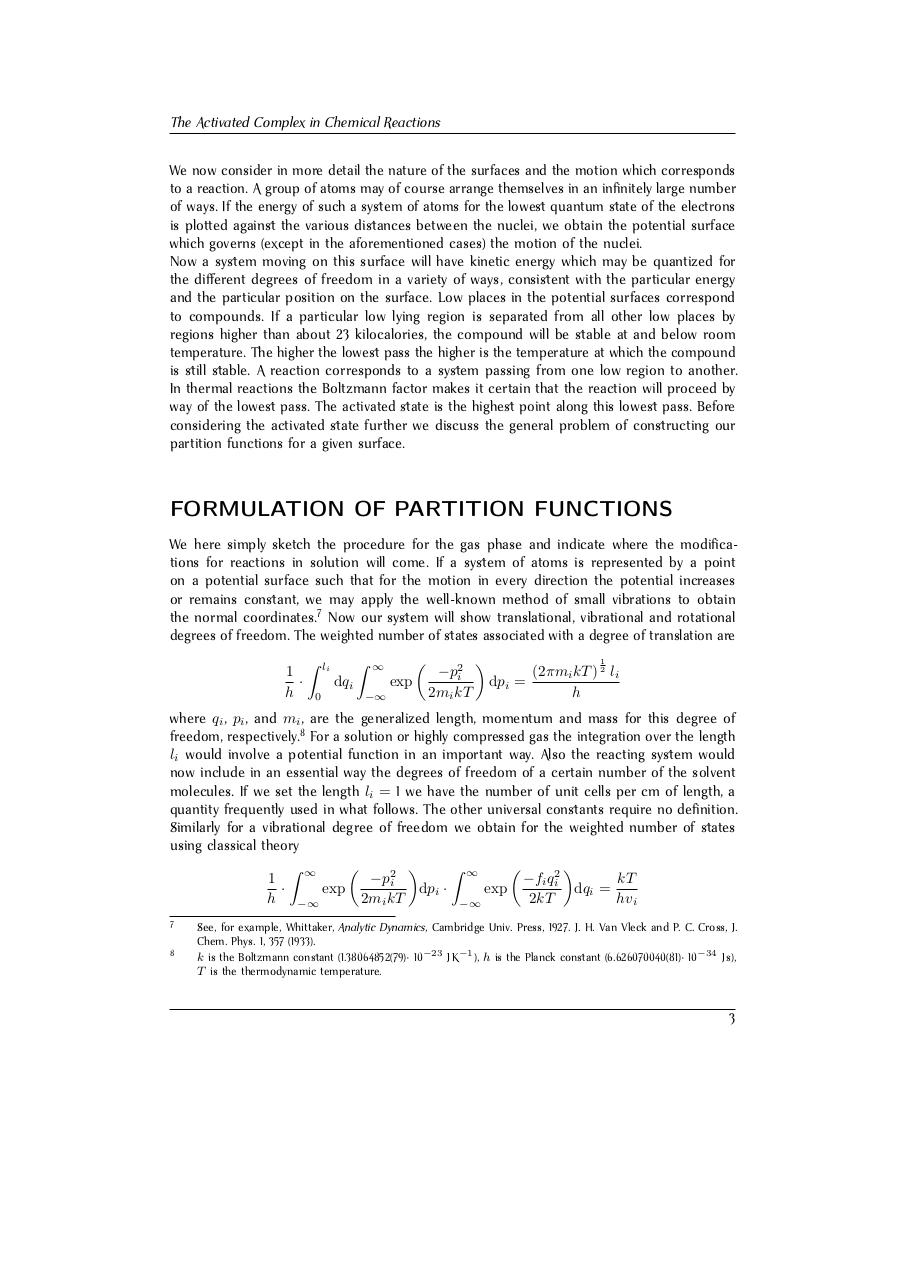# Henry Eyring. The Activated Complex in Chemi.pdfPage 1 2 3 4 5 6 7 8 9 10 11 12 13

#### Text preview

The Activated Complex in Chemical Reactions
We now consider in more detail the nature of the surfaces and the motion which corresponds
to a reaction. A group of atoms may of course arrange themselves in an infinitely large number
of ways. If the energy of such a system of atoms for the lowest quantum state of the electrons
is plotted against the various distances between the nuclei, we obtain the potential surface
which governs (except in the aforementioned cases) the motion of the nuclei.
Now a system moving on this surface will have kinetic energy which may be quantized for
the different degrees of freedom in a variety of ways, consistent with the particular energy
and the particular position on the surface. Low places in the potential surfaces correspond
to compounds. If a particular low lying region is separated from all other low places by
regions higher than about 23 kilocalories, the compound will be stable at and below room
temperature. The higher the lowest pass the higher is the temperature at which the compound
is still stable. A reaction corresponds to a system passing from one low region to another.
In thermal reactions the Boltzmann factor makes it certain that the reaction will proceed by
way of the lowest pass. The activated state is the highest point along this lowest pass. Before
considering the activated state further we discuss the general problem of constructing our
partition functions for a given surface.

FORMULATION OF PARTITION FUNCTIONS
We here simply sketch the procedure for the gas phase and indicate where the modifications for reactions in solution will come. If a system of atoms is represented by a point
on a potential surface such that for the motion in every direction the potential increases
or remains constant, we may apply the well-known method of small vibrations to obtain
the normal coordinates.7 Now our system will show translational, vibrational and rotational
degrees of freedom. The weighted number of states associated with a degree of translation are
1
·
h

Z

li

Z

dqi
0


exp

−∞

−p2i
2mi kT

1


dpi =

(2πmi kT ) 2 li
h

where qi , pi , and mi , are the generalized length, momentum and mass for this degree of
freedom, respectively.8 For a solution or highly compressed gas the integration over the length
li would involve a potential function in an important way. Also the reacting system would
now include in an essential way the degrees of freedom of a certain number of the solvent
molecules. If we set the length li = 1 we have the number of unit cells per cm of length, a
quantity frequently used in what follows. The other universal constants require no definition.
Similarly for a vibrational degree of freedom we obtain for the weighted number of states
using classical theory




Z ∞
Z ∞
1
−p2i
kT
−fi qi2
·
exp
dpi ·
dqi =
exp
h −∞
2mi kT
2kT
hv
i
−∞
7
8

See, for example, Whittaker, Analytic Dynamics, Cambridge Univ. Press, 1927. J. H. Van Vleck and P. C. Cross, J.
Chem. Phys. 1, 357 (1933).
k is the Boltzmann constant (1.38064852(79)· 10−23 J K−1 ), h is the Planck constant (6.626070040(81)· 10−34 J s),
T is the thermodynamic temperature.

3